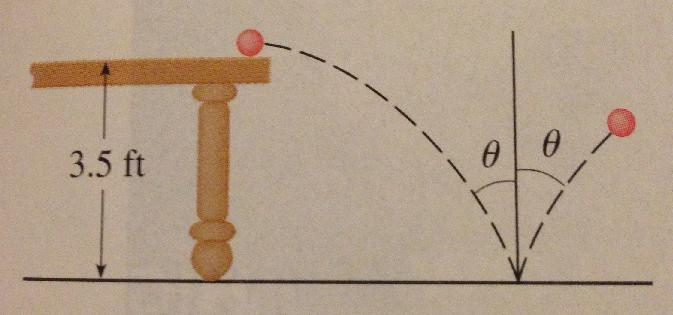# How to: toss a ball into the bucketA ball rolls off a table with a speed of $2ft/s$. The table is $3.5$ ft high.

Suppose the ball rebounds from the floor at the same angle with which it hits the floor, but loses $20$% of its speed due to energy absorbed by the ball on impact. Where does the ball strike the floor on the second bounce?

Enter the answer as the displacement of the ball in feet to the right of the table's edge.

Details and Assumptions:

• $g=32.1740ft/s^2$
• The table is perfectly horizontally flat (and so is the ground) - the picture is not drawn to scale.
• Round the answer to the nearest hundredth
×